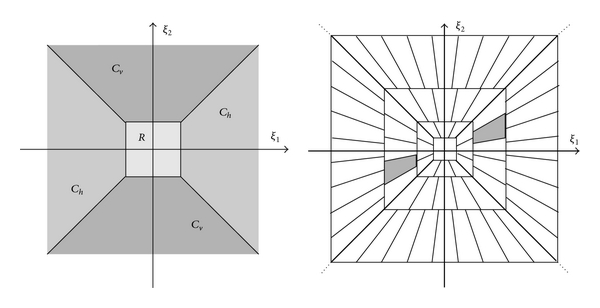## Shearlet Systems

A shearlet system enables you to create directionally sensitive sparse representations of images with anisotropic features. Shearlets are used in image processing applications including denoising, compression, restoration, and feature extraction. Shearlets are also used in statistical learning to address problems of image classification, inverse scattering problems such as tomography, and data separation. You can find additional applications at ShearLab .

A strength of wavelet analysis for 1-D signals is its ability to efficiently represent smooth functions that have pointwise discontinuities. However, wavelets do not represent curved singularities, such as the edge of a disk in an image, as sparsely as they do pointwise discontinuities. Geometric multiscale analysis is an attempt to design systems capable of efficiently representing curved singularities in higher dimensional data. In addition to shearlets, other geometric multiscale systems include curvelets, contourlets, and bandlets.

Guo, Kutyniok, and Labate  pioneered the development of the theory of shearlets. They. also developed efficient algorithms for shearlet transforms , as have Häuser and Steidl . ShearLab  provides an extensive set of algorithms for processing two- and three-dimensional data using shearlets.

Like wavelets, a comprehensive theory relates the continuous shearlet transform with the discrete transform. Also, a multiresolution analysis framework exists for shearlets. As the name suggests, shearlets have the noteworthy feature of using shears, not rotations, to control directional sensitivity. This characteristic allows you to create a shearlet system from a single or finite set of generating functions. Other reasons for the success of shearlets include:

• Shearlets provide optimally sparse approximations of anisotropic features of multivariate data.

• Both compactly supported and bandlimited shearlets exist.

• Shearlet transforms have efficient algorithmic implementations.

### Shearlets

Similar to wavelets, shearlets do not have a unique system. Dilation, shearing, and translation operations generate the shearlets. A dilation can be expressed as a matrix, ${A}_{a}=\left(\begin{array}{cc}{a}^{1/2}& 0\\ 0& {a}^{-1/2}\end{array}\right)$ where $a\in {ℝ}^{+}.$ A shear can be expressed as ${S}_{s}=\left(\begin{array}{cc}1& s\\ 0& 1\end{array}\right)$ where $s\in ℝ.$ The variable s parameterizes orientations.

If the function $\psi \in {L}^{2}\left({ℝ}^{2}\right)$ satisfies certain (admissibility) conditions, then the set of functions

`$SH\left\{\psi \right\}=\left\{{\psi }_{a,s,t}={a}^{3/4}\psi \left({S}_{s}{A}_{a}\left(·-t\right)\right)\right\}$`

is a continuous shearlet system where a and s are defined as noted earlier, and $t\in {ℝ}^{2}.$

If you discretize the dilation, shearing, and translation parameters appropriately, you obtain a discrete shearlet system:

`$SH\left(\psi \right)=\left\{{\psi }_{j,k,m}={2}^{3}{4}j}\psi \left({S}_{k}{A}_{{2}^{j}}·-m\right):j,k\in ℤ,m\in {ℤ}^{2}\right\}.$`

The function `shearletSystem` creates a cone-adapted bandlimited shearlet system. The implementation of the `shearletSystem` function follows the approach described in Häuser and Steidl . The shearlet system is an example of a frame, which you can normalize to create a Parseval frame. The discrete shearlet transform of a function $f\in {L}^{2}\left({ℝ}^{2}\right)$ is the inner product of $f$ with all the shearlets in the discrete shearlet system $〈f,{\psi }_{j,k,m}〉$ where $\left(j,k,m\right)\in ℤ×ℤ×{ℤ}^{2}.$ You use `sheart2` to take the discrete shearlet transform of an image. For additional information, see the References.

The following figure shows how a cone-adapted shearlet system partitions the 2-D frequency plane. The image on the left shows the partition of a cone-adapted real-valued shearlet system with one scale. The R region in the center is the lowpass part of the system. In addition, the image includes a horizontal cone shearlet (symmetric in frequency because it is real valued) and a vertical cone shearlet. The image on the right depicts a system with three scales. The fan-like pattern gives a shearlet system its directional sensitivity. Note that the number of shearing factors increases as the frequency support of the shearlet increases. As the support in the frequency domain increases, the support in the spatial domain decreases.The spectra of the real-valued shearlets are the same over the positive and negative ξ1, ξ2 supports. Shearlets in complex-valued shearlet systems partition individually, not in pairs.

### Transform Type

Shearlets are either real valued or complex valued in the spatial domain. You specify the transform type when you use `shearletSystem` to create the system. Real-valued shearlets have two-sided frequency spectra. The Fourier transforms of the complex-valued shearlets have support on only one half of the 2-D frequency space. The Fourier transforms of both types of shearlets are real valued.

### References

 Guo, K., G. Kutyniok, and D. Labate. "Sparse multidimensional representations using anisotropic dilation and shear operators." Wavelets and Splines: Athens 2005 (G. Chen, and M.-J. Chen, eds.), 189–201. Brentwood, TN: Nashboro Press, 2006.

 Guo, K., and D. Labate. "Optimally Sparse Multidimensional Representation Using Shearlets." SIAM Journal on Mathematical Analysis. Vol. 39, Number 1, 2007, pp. 298–318.

 Kutyniok, G., and W.-Q Lim. "Compactly supported shearlets are optimally sparse." Journal of Approximation Theory. Vol. 163, Number 11, 2011, pp. 1564–1589.

 Shearlets: Multiscale Analysis for Multivariate Data (G. Kutyniok, and D. Labate, eds.). New York: Springer, 2012.

 ShearLab. `https://www3.math.tu-berlin.de/numerik/www.shearlab.org/`.

 Häuser, S., and G. Steidl. "Fast Finite Shearlet Transform: a tutorial." arXiv preprint arXiv:1202.1773 (2014).

 Rezaeilouyeh, H., A. Mollahosseini, and M. Mahoor. "Microscopic medical image classification framework via deep learning and shearlet transform." Journal of Medical Imaging. Vol. 3, Number 4, 044501, 2016. doi:10.1117/1.JMI.3.4.044501.